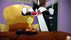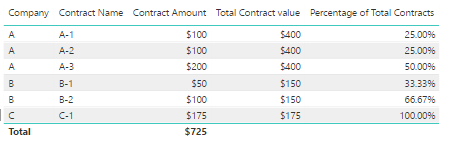cancel
Showing results for
Did you mean:Helper II

## Percent of Column Total

Hello,

In what would apparently seem like a staightforward thing to do, I am trying to capture the percent of each contract with a customer to the total contract amount with said customer. For example, customer A has three contracts, each for \$100,000. The total for that customer is \$300,000 and the percentage of each contract is 33.33%. I have tried the following DIVIDE(sum('Contract'[Net Contract Amount]), CALCULATE([Net Contract Amount], ALLSELECTED('Customer'[CustomerID]))). The value that comes back for each row is 100%. There is somethign amiss with my context since the only way you can get to 100% for each row is that the measure is dividing each row by itselt: \$100,000 divided by \$100,000 equals 1.  The measure is to be used primarily in a table. Thanks for your assistance.

1 ACCEPTED SOLUTIONResponsive Resident
`Percent of Total = DIVIDE(sum('Contract'[ContractAmount], RELATED('Vendor'[Vendor Total]), 0)`

Take out that sum. You don't need it.

`Percentage of Total Contracts = divide([Contract Amount],related('Companies'[Total Contract Value]),0)`
14 REPLIES 14Anonymous
Not applicable

I am having difficulty replicating the formula.

I have a GBP value column [Settlement] and a text value colum [Channel].

I need to see what percentage of the total [Settlement] is for each [Channel]

Thanks

DomSuper User

Just use the "Show value as" | "Percent of grand total"?

Click the little arrow drop down on your value column in the Visualizations area and choose those menu options.

Become an expert!: Enterprise DNA
External Tools: MSHGQM
Latest book!:
Mastering Power BI 2nd EditionDAX is easy, CALCULATE makes DAX hard...Helper II

I need to store a value since I have to then multiply that percentage against another value. In this example, the customer has a credit limit of \$750,000, so each row in the table needs to have the percentage multiplied by the credit limit.Super User

OK, what you probably want is an ALLEXCEPT clause instead of ALLSELECTED. Sample data please.

Become an expert!: Enterprise DNA
External Tools: MSHGQM
Latest book!:
Mastering Power BI 2nd EditionDAX is easy, CALCULATE makes DAX hard...Helper II
 Contract Number VendorID Contract Amount Pct of Total 7223DIAM 49113 \$959,574 41.99% 7239DIAM 49113 \$805,790 35.26% 7351DIAM 49113 \$519,670 22.74% \$2,285,034 100.00%

The percent of total is a simple formula in Excel. The percentages will subsequently be used in another measure/calculation. If I use ALLEXCEPT, all except what? The VendorID is the primary key in the vendor table and subcontract number is a dependency.Super User

ALLEXCEPT whatever filters you are trying to preserve. So, in the case below you would probably just need to use VendorID I believe.

Become an expert!: Enterprise DNA
External Tools: MSHGQM
Latest book!:
Mastering Power BI 2nd EditionDAX is easy, CALCULATE makes DAX hard...Responsive Resident

I created a table with just the companies and then added column based on this:

`Total Contract value = sumx(Calculatetable('Contracts',filter('Contracts',[Company]='Companies'[Company])),[Contract Amount])`

Then on the Contracts table I created another column based on this:

`Percentage of Total Contracts = [Contract Amount]/related('Companies'[Total Contract Value])`

Which gave the following results:I can let you have the PBIX file if you would like.Anonymous
Not applicable

Greetings

Can you kindly share the file pleaseHelper II

When I go to calculate the percentage, I get a DAX error: "The column either doesn't exist or doesn't have a relationship to any table available in the current context." I do have a relationship defined between the contract table and the vendor table. The contract table is the "many" side of the relationship. I do know that the total amount at the vendor level is correct. Just so I'm clear, the percent of total is a column as opposed to a measure, correct?Responsive Resident

Just so I'm clear, the percent of total is a column as opposed to a measure, correct?

Yes, both of these were columns.Responsive Resident

What does your formula look like?Helper II

Percent of Total = DIVIDE(sum('Contract'[ContractAmount], RELATED('Vendor'[Vendor Total]), 0)

I also have a measure defined in the contracts table Contract Amount = sum('Contract'[ContractAmount])

On the vendor side, I have SUMX(CALCULATETABLE('Contract', FILTER('Contract', [VendorID] =  'Vendor'[VendorID])), [Contract Amount])Responsive Resident
`Percent of Total = DIVIDE(sum('Contract'[ContractAmount], RELATED('Vendor'[Vendor Total]), 0)`

Take out that sum. You don't need it.

`Percentage of Total Contracts = divide([Contract Amount],related('Companies'[Total Contract Value]),0)`Helper II

That did it! Thank you!!Announcements#### Exclusive opportunity for Women!

Join us for a free, hands-on Microsoft workshop led by women trainers for women where you will learn how to build a Dashboard in a Day!#### Power Platform Conference-Power BI and Fabric Sessions

Join us Oct 1 - 6 in Las Vegas for the Microsoft Power Platform Conference.Top Solution Authors
Top Kudoed Authors
Users online (1,598)